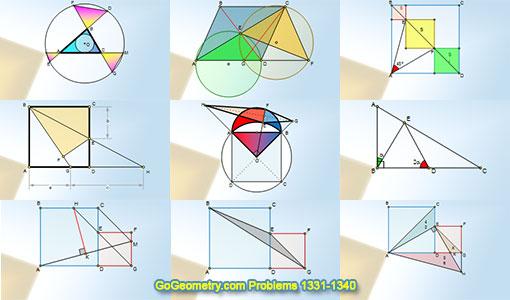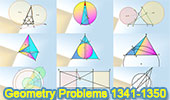# Geometry Problems 1341-1350. Level: High School, CollegeGeometry Problems 1341 - 1350Problem 1341. Isosceles Triangle, 80-20-80 Degrees, Circumcenter, Angle Bisector. Problem 1342. Circle, Secant, Chord, Midpoint, Concyclic Points, Cyclic Quadrilateral. Problem 1343. Triangle, Excircles, Circle, Tangent, Tangency Points, Chord, Perpendicular, 90 Degrees, Collinearity. Machu Picchu in the background. Geometry Problem 1344, Bottema's Theorem: Triangle and Squares with Interactive Geometry Software. Geometry Problem 1345: Three Equilateral Triangles, Center, Area, Common Vertex. Geometry Problem 1346.Equilateral Triangle, Point on the Incircle, Altitude, Perpendicular, Sum of Squares, Distance. Geometry Problem 1347.Triangle, Angle Bisector, Congruence, Circumcircle, Tangent, Equilateral Triangle. Geometry Problem 1348.Three Concentric Circles, Equilateral Triangle, Perpendicular Bisector, Congruence. Geometry Problem 1349.Three Squares, 90 Degrees, Perpendicular Lines, Diagonal. Math Infographic. Geometry Problem 1350.Triangle with three Intersecting Circles, Incircle, Collinear Points.

Home | Sitemap | Geometry | Problems | Visual Index | 10 ProblemsEmail | Post a comment | by Antonio Gutierrez
Last updated: Jul 11, 2018